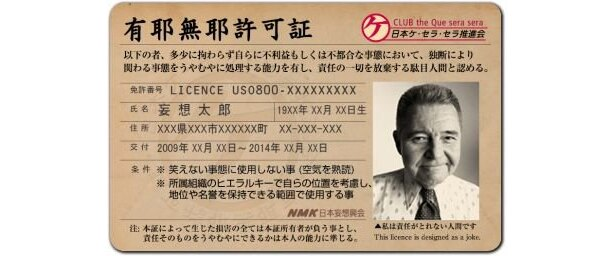# 日本人說的「有耶無耶」到底是有還是沒有？Image source: www.walkerplus.com/article/11984/image39940.html

「有耶無耶」是日文的四字熟語。如果用中文的角度來看，「耶」在文言文中相當於疑問詞的「嗎？」、「呢？」，例如「然則何時而樂耶?」。如果把有耶無耶，解讀成「有嗎？沒有嗎？」，那到底是有還是沒有啊？其實，重點是在字面以外的弦外之音。以下解釋完日文的部分之後，我會再加碼解說英文的類似用語。

## 有耶無耶 = うやむや = 有 (あ)るか無 (ない)いか曖昧 (あいまい)

あの (けん)はうやむやになった。

👉

👉

👉

## 英文表達「曖昧又模糊」的幾種說法

### leave… unanswered (liv… ˌʌˈnænsərd) / leave… unsettled (liv… ənˈsɛtəld)

The mayor (((市長))) left the question about his scandal (((醜聞))) unanswered in a press meeting (((記者會))) .

The government (((政府))) is leaving the accusation (((指控))) by an unnamed (((不具名的))) informant (((線民))) unsettled.

### cloud (klaʊd)

He had a hangover (((宿醉))) waking up (((起床))) this morning (((早上))) and found (((發現))) he couldn’t remember (((記得))) a thing (((事情))) about last night (((昨晚))) because (((因為))) alcohol (((酒精))) had clouded his memory (((記憶))) .

When (((當))) lives (((人命))) are at stake (((處於危險))) , the president (((總統))) must (((必須))) not allow (((允許))) politics (((政治))) to cloud his judgment (((判斷))) .。

### ambiguous (æmˈbɪgjuəs) / ambiguity (ˌæmbɪˈgjuəti)

Don’t give (((給))) me an ambiguous (()) attitude (((態度))) like (((像))) that!

There seems to be (((似乎有))) ambiguity (((模糊))) in our discussion (((討論))) . Maybe (((或許))) we should (((應該))) clear things up (((澄清事情))) .

### vague (veɪg)

vague的語感就好像眼鏡被遮上了一層霧氣，或是近視很重卻找不到眼鏡時看著四周都很模糊的樣子。形容詞vague是講模糊的狀態，也可拿來形容曖昧模糊不清。

His attitude on (((在…方面))) this matter (((事情))) is a little (((有點))) vague.他對這件事的態度有點曖昧不清。

You shouldn’t be vague about her feelings (((感情))) for you. Just tell (((告訴))) her if (((如果))) you like her or not.